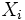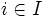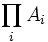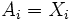Hausdorffness is product-closed

This article gives the statement, and possibly proof, of a topological space property (i.e., Hausdorff space) satisfying a topological space metaproperty (i.e., product-closed property of topological spaces)
View all topological space metaproperty satisfactions | View all topological space metaproperty dissatisfactions
Get more facts about Hausdorff space |Get facts that use property satisfaction of Hausdorff space | Get facts that use property satisfaction of Hausdorff space|Get more facts about product-closed property of topological spaces

Statement

Verbal statement

An arbitrary (finite or infinite) product of Hausdorff spaces, when endowed with the product topology, is also a Hausdorff space.

Definitions used

Hausdorff space

Further information: Hausdorff space

A topological space$X$ is Hausdorff if given distinct points$a,b \in X$, there exist disjoint open sets$U, V$ containing$a$ and$b$.

Product topology

Further information: Product topology

Suppose$I$ is an indexing set, and$X_i$ a family of topological spaces,$i \in I$. Then if$X$ is the Cartesian product of the$X_i$s, the product topology on$X$ is a topology with subbasis given by all the open cylinders: all sets of the form$\prod_i A_i$ such that for all but one$i$,$A_i = X_i$, and for the one exceptional$i$,$A_i$ is an open subset of$X_i$.

Proof

Proof outline

The proof has the following three steps:

• Write down both points in the product space as tuples
• Find a coordinate where they differ, and separate the projections on that coordinate, by disjoint open sets in that coordinate (this is where we use that each space is Hausdorff)
• Use the open cylinders corresponding to these disjoint open subsets, to separate the original two points